#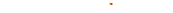###### Scale

Basics

Scale Type

 Constant Fix the size to keep it the same Newton Dynamics Set the size based on values speed and acceleration Curve Value Set the fixed size for keyframes in Timeline Curve Speed Set the scale speed for keyframes in Timeline

Constant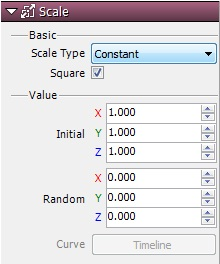Set the initial size and from there make the selection so that it won’t move. Compared to other function types, one characteristic of this function is the light processing load on the CPU.

Newton Dynamics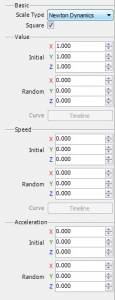In addition to adding values, movement speed and acceleration can be set.

 Value Specify the initial sizes of particles. The random value is initial scale with a random range. The random range is between -(specified value) and +(specified value). Speed Specify the scale speeds for particles. The random value is speed with a random range. The random range is between -(specified value) and +(specified value). Acceleration Specify the scale accelerations for particles. The random value is acceleration with a random range. The random range is between -(specified value) and +(specified value).

Curve Value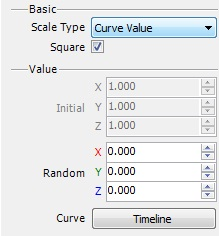Set the scale values for graphs in Timeline. Change keyframes in real time as they run.

Curve Speed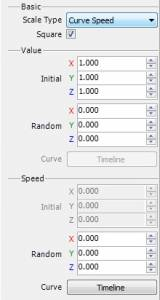Set the speed values to scale for graphs with Timeline. Change keyframes in real time as they run.